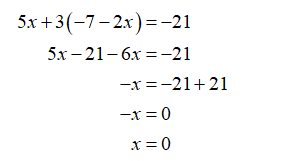Question
1 views

Solve by substitution:

2x + y = − 7
5x + 3y = − 21

check_circle

Step 1

Here,

2x + y = − 7 ….(1)
5x + 3y = − 21 ….(2)

Step 2

Derive the equation of y from equation (1)

2x+y = -7

y= -7-2x

Step 3

Substitute it in the equation (2)....

### Want to see the full answer?

See Solution

#### Want to see this answer and more?

Solutions are written by subject experts who are available 24/7. Questions are typically answered within 1 hour.*

See Solution
*Response times may vary by subject and question.
Tagged in